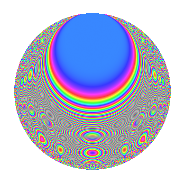# Properties

 Label 150.2.lLevel 150 Weight 2 Character orbit l Rep. character $$\chi_{150}(17,\cdot)$$ Character field $$\Q(\zeta_{20})$$ Dimension 80 Newforms 1 Sturm bound 60 Trace bound 0

# Related objects

## Defining parameters

 Level: $$N$$ = $$150 = 2 \cdot 3 \cdot 5^{2}$$ Weight: $$k$$ = $$2$$ Character orbit: $$[\chi]$$ = 150.l (of order $$20$$ and degree $$8$$) Character conductor: $$\operatorname{cond}(\chi)$$ = $$75$$ Character field: $$\Q(\zeta_{20})$$ Newforms: $$1$$ Sturm bound: $$60$$ Trace bound: $$0$$

## Dimensions

The following table gives the dimensions of various subspaces of $$M_{2}(150, [\chi])$$.

Total New Old
Modular forms 272 80 192
Cusp forms 208 80 128
Eisenstein series 64 0 64

## Trace form

 $$80q$$ $$\mathstrut +\mathstrut 4q^{3}$$ $$\mathstrut +\mathstrut 4q^{7}$$ $$\mathstrut +\mathstrut O(q^{10})$$ $$80q$$ $$\mathstrut +\mathstrut 4q^{3}$$ $$\mathstrut +\mathstrut 4q^{7}$$ $$\mathstrut -\mathstrut 4q^{10}$$ $$\mathstrut -\mathstrut 4q^{12}$$ $$\mathstrut -\mathstrut 8q^{15}$$ $$\mathstrut +\mathstrut 20q^{16}$$ $$\mathstrut -\mathstrut 8q^{18}$$ $$\mathstrut -\mathstrut 40q^{19}$$ $$\mathstrut -\mathstrut 36q^{22}$$ $$\mathstrut -\mathstrut 104q^{25}$$ $$\mathstrut +\mathstrut 4q^{27}$$ $$\mathstrut -\mathstrut 16q^{28}$$ $$\mathstrut +\mathstrut 12q^{30}$$ $$\mathstrut +\mathstrut 4q^{33}$$ $$\mathstrut -\mathstrut 40q^{34}$$ $$\mathstrut -\mathstrut 24q^{37}$$ $$\mathstrut -\mathstrut 40q^{39}$$ $$\mathstrut -\mathstrut 8q^{40}$$ $$\mathstrut -\mathstrut 4q^{42}$$ $$\mathstrut -\mathstrut 24q^{43}$$ $$\mathstrut -\mathstrut 72q^{45}$$ $$\mathstrut -\mathstrut 4q^{48}$$ $$\mathstrut -\mathstrut 12q^{55}$$ $$\mathstrut -\mathstrut 64q^{57}$$ $$\mathstrut +\mathstrut 20q^{58}$$ $$\mathstrut +\mathstrut 24q^{60}$$ $$\mathstrut +\mathstrut 64q^{63}$$ $$\mathstrut +\mathstrut 96q^{67}$$ $$\mathstrut +\mathstrut 140q^{69}$$ $$\mathstrut +\mathstrut 76q^{70}$$ $$\mathstrut +\mathstrut 8q^{72}$$ $$\mathstrut +\mathstrut 100q^{73}$$ $$\mathstrut +\mathstrut 132q^{75}$$ $$\mathstrut +\mathstrut 100q^{78}$$ $$\mathstrut +\mathstrut 80q^{79}$$ $$\mathstrut -\mathstrut 40q^{81}$$ $$\mathstrut +\mathstrut 96q^{82}$$ $$\mathstrut +\mathstrut 60q^{84}$$ $$\mathstrut +\mathstrut 32q^{85}$$ $$\mathstrut +\mathstrut 80q^{87}$$ $$\mathstrut +\mathstrut 4q^{88}$$ $$\mathstrut +\mathstrut 52q^{90}$$ $$\mathstrut +\mathstrut 12q^{93}$$ $$\mathstrut -\mathstrut 32q^{97}$$ $$\mathstrut +\mathstrut O(q^{100})$$

## Decomposition of $$S_{2}^{\mathrm{new}}(150, [\chi])$$ into irreducible Hecke orbits

Label Dim. $$A$$ Field CM Traces $q$-expansion
$$a_2$$ $$a_3$$ $$a_5$$ $$a_7$$
150.2.l.a $$80$$ $$1.198$$ None $$0$$ $$4$$ $$0$$ $$4$$

## Decomposition of $$S_{2}^{\mathrm{old}}(150, [\chi])$$ into lower level spaces

$$S_{2}^{\mathrm{old}}(150, [\chi]) \cong$$ $$S_{2}^{\mathrm{new}}(75, [\chi])$$$$^{\oplus 2}$$